Next: Hartree-Fock method Up: MANY-NUCLEON SYSTEMS Previous: Effective Interactions (I)

Effective Interactions (II)

To a certain extent, a way out from the explosion of dimensionality, discussed in Sec. 4.1, may consist in using a better single-particle space. Instead of parametrizing fields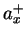by space-spin-isospin points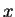, one can use a parametrization by the shell-model orbitals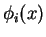that are active near the Fermi surface of a given nucleus, i.e., by fields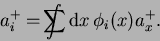(64)

When a complete set of orbitals is used, the descriptions in terms of creation operators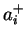andare equivalent. However, one can also attempt a drastic reduction of the setto a finite number,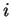=1...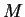, of most important'' orbitals, similarly as we have been previously using finite sets of the space-spin-isospin points instead of continuous variables.

The reduction is now not a mere question of discretizing continuous fields, but involves a serious limitation of the Hilbert space. In quantum mechanics one can always split the Hilbert space into two subspaces,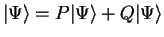, where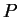and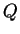are projection operators such that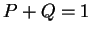. Then, the Schrödinger equation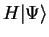=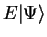is strictly equivalent to the following 2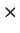2 matrix of equations,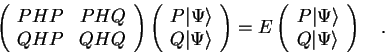(65)

Using the second equation, one can now formally express the excluded'' component,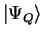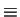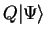, of the wave function by the kept'' component,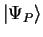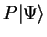, i.e.,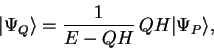(66)

and put it back into the first equation. This gives the Schrödinger equation reduced to the kept'' Hilbert space,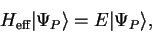(67)

where the effective Hamiltonian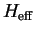is given by the Bloch-Horowitz equation Blo58,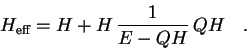(68)

The main questions is, of course, whether the Bloch-Horowitz effective interaction,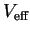=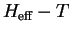, can be replaced by a simple phenomenological interaction, and used to describe real systems. In particular, when a two-body, energy-independent interaction is postulated in a very small phase space, one obtains the shell model, which is successfully used since many years in nuclear structure physics.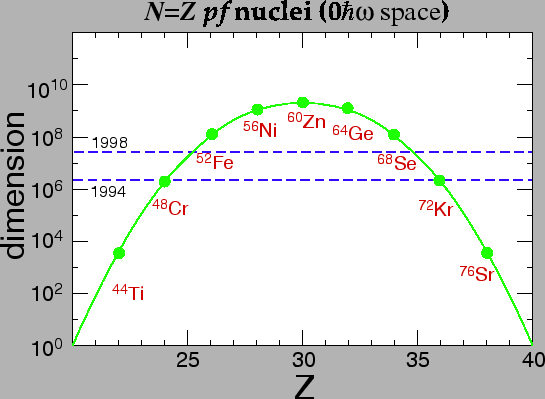In order to illustrate the dimensions of the shell-model Hilbert space, in Fig. 9 we show the numbers of many-fermion states that are obtained when states in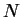=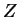medium heavy nuclei are described within the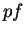space (20 s.p. states for protons and 20 for neutrons). Currently, complete solutions for thespace become available, i.e., dimensions of the order of 10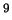can effectively be treated. Progress in this domain closely follows the progress in size and speed of computers, i.e., one order of magnitude is gained in about every two-three years. We shell not discuss these methods in any more detail, because dedicated lectures have been presented on this subject during the Summer School.Next: Hartree-Fock method Up: MANY-NUCLEON SYSTEMS Previous: Effective Interactions (I)
Jacek Dobaczewski 2003-01-27